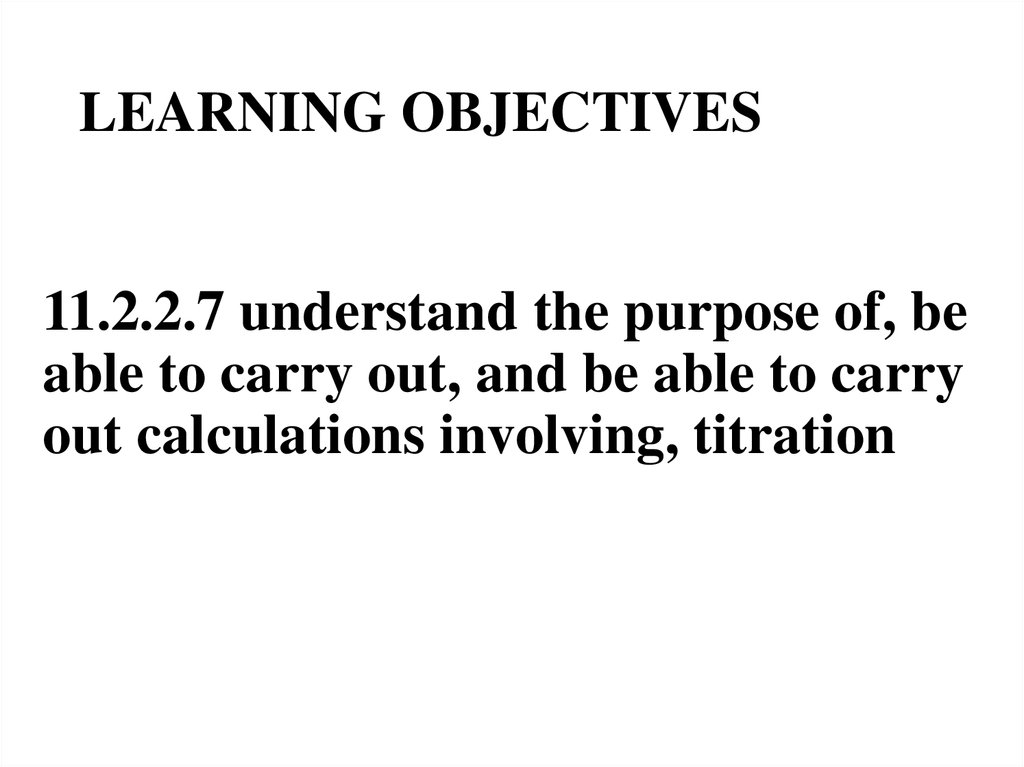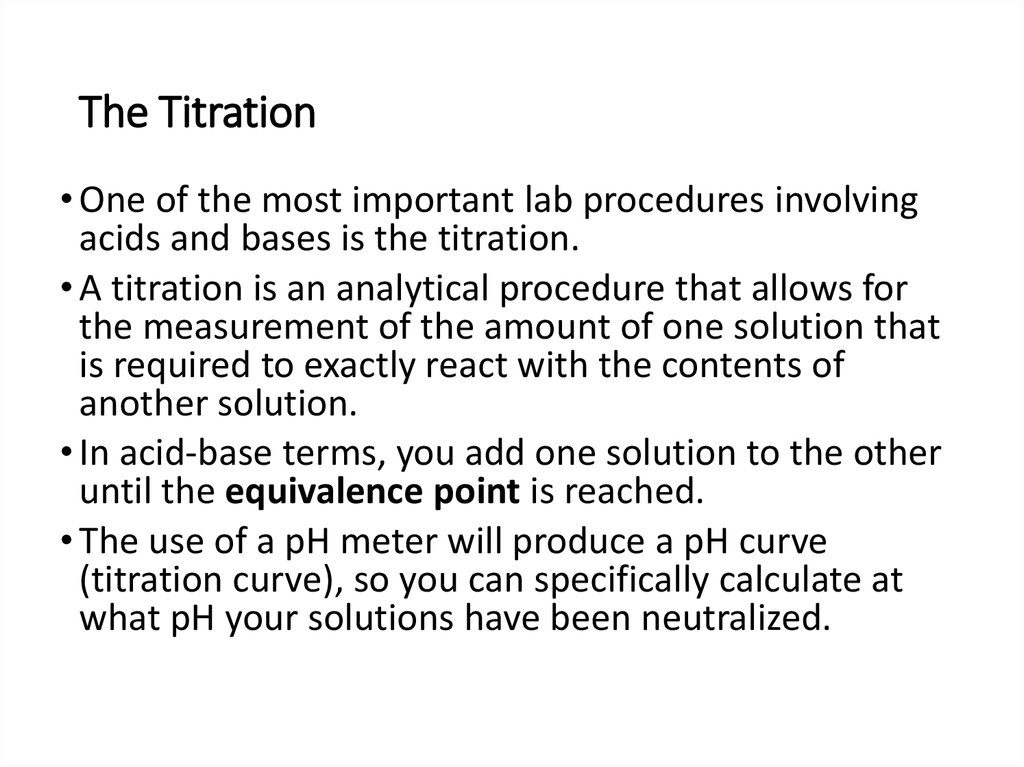# Titration and Acid-Base Neutralization

## 2. LEARNING OBJECTIVES

11.2.2.7 understand the purpose of, be
able to carry out, and be able to carry
out calculations involving, titration

## 3. The Titration

• One of the most important lab procedures involving
acids and bases is the titration.
• A titration is an analytical procedure that allows for
the measurement of the amount of one solution that
is required to exactly react with the contents of
another solution.
• In acid-base terms, you add one solution to the other
until the equivalence point is reached.
• The use of a pH meter will produce a pH curve
(titration curve), so you can specifically calculate at
what pH your solutions have been neutralized.

## 4. Acid-Base Titration Terms to Know

• Titrant: the standard solution of known molarity in the buret that is being added
to the solution in the flask. This is more often the acid than the base.
• Analyte: the solution in the flask of unknown concentration. Usually the base.
• Indicator: a compound that is added in small amounts (a few drops) in acid-base
titrations. It changes color over a certain pH range, and indicates the end of the
titration. This range should be matched with pH at which you expect your
solutions to reach the equivalence point.
• Endpoint: the point at which the titration is stopped, when the indicator
permanently changes color. Traditionally, this is the point when the titration is
stopped, where the number of moles of titrant is equal to the number of moles
of analyte, or some multiple thereof (as in di- or tri- protic acids)
• Equivalence point (a.k.a. neutralization or endpoint): the point (in mL of solution
added) at which the number of moles of acid equal the number of moles of
base.

## 5. Types of Acid-Base Titrations

• The quality of the titration depends on the strength of the acids and
bases you use. This, in turn, will affect the resulting pH curve.
• Let’s look at a few examples of pH curves.

## 6. Strong Acid-Strong Base

• This diagram shows the change in
pH as a solution of strong acid is
slowly added to a solution of
strong base.
• Strong acid-strong base titrations
usually have an equivalence point
around 7.
• This is due to the fact that in
solution, strong acids and strong
bases will both completely
dissociate, so there is an
approximate 1:1 ratio of moles of
acid to moles of base at the
equivalence point.

## 7. Strong Base-Strong Acid

• Strong base-strong acid titrations are just like the strong acidstrong base titrations: the pH at the equivalence point is
around 7
• Note that in both types, there is relatively little change in pH
until it jumps steeply at the equivalence point.

## 8. Titration Equipment

• Stand
• Buret
• Buret clamp
shows a beaker)
• Analyte (unknown molarity)
• Titrant (standard solution of
known molarity)
• Indicator
• pH meter (optional, but highly
recommended)
• Stirrer (optional, recommended)

## 9. Preparing the Titration

• Make sure your equipment is clean!
• Take care when preparing the buret. Run distilled water through it to
make sure it is clean. Then, rinse it with some of the titrant solution,
letting it run through to stopcock as well. This will ensure that there
is no water left, so that the concentration of the titrant is not
unexpectedly diluted when you actually perform the titration.
• Measure out the volume of the analyte that you add to the flask, and
record it. Add a few drops of an appropriate indicator. Set the flask on
the stirrer and the magnet inside in flask.
• Fill the buret with an appropriate amount of titrant, and record this
initial amount. Secure the buret to the stand over the flask with a
clamp.
• Put the pH meter in the flask, and secure it with a clamp.

## 10. Starting the Titration

• Turn the stirrer onto a low setting.
• Add a few milliliters of titrant to the
analyte at a time by switching the
stopcock between the open and
closed positions, and record the pH
• As the titrant is dropped into the
analyte, the indicator will briefly
change color, and then disappear.
• The initial changes in pH will be very
small, since all of the added titrant
will be reacted by the excess analyte

## 11. Around the Endpoint

• You have reached the
endpoint when the
indicator first
permanently changes
color.
• There will be a very large
jump in pH as all of the
analyte is reacted, and
there is now excess
titrant.
Example of a solution using
phenolphthalein as a indicator. The
reaction has just reached the equivalence
point, because the solution has just
permanently turned pale pink.

## 12. Acid Base Neutralization Reaction

•Acid
+ Base Water + Salt
•Ex: HCl + NaOH H2O + NaCl

## 14. Titration:

•A laboratory method for
determining the concentration of an
unknown acid or base using a
neutralization reaction.
•A standard solution,(a solution of
known concentration), is used.

## 15. Equivalence Point

•The point at which there are
stoichiometrically equivalent
amounts of acid and base.
•[H+] = [OH-]

Buret
Valve

Acid with
Phenolpthalein
End-Point

## 19. Indicators

• Indicators are chosen, such that they change
colors at the range of the pH of interest.
• The solution itself at the end-point may be:
• Basic, if the reaction involves a strong base
and a weak acid.
• Neutral, if the reaction involves a strong acid
and a strong base.
• Acidic, if the reaction involves a strong acid
and a weak base.

## 20. Methods of Solving Titration Problems:

a) using stoichiometry
b) using the titration formula
aMaVa=bMbVb.

## 21. Ex. 1

What is the concentration of HCl if 30.0
mL of 0.10 M NaOH neutralizes 50.0mL
HCl?
NaOH + HCl H2O + NaCl
Hint: Use aMaVa=bMbVb
Ma=
How many moles of HCl were used?
Hint: #moles= MaVa , but convert the
volume to L( 50mL=0.05L).

## 22. Ex. 2

A 20.0 mL solution of Sr(OH)2 is
neutralized after 25.0 mL of
standard 0.05 M HCl is added. What
is the concentration of Sr(OH)2?
2 HCl + Sr(OH)2 2 H2O + SrCl2

## 23. Ex. 3

•How many mL of 0.20 M H3PO4 are
needed to neutralize 55.0 mL of a
0.10 M solution of NaOH?

## 24. Ex. 4

•What volume of 0.20M Ca(OH)2 will
neutralize 45.0 mL of a 1M solution
of HCl?